# WBJEE Maths Test - 10

## 75 Questions MCQ Test WBJEE Sample Papers, Section Wise & Full Mock Tests | WBJEE Maths Test - 10

Description
Attempt WBJEE Maths Test - 10 | 75 questions in 120 minutes | Mock test for JEE preparation | Free important questions MCQ to study WBJEE Sample Papers, Section Wise & Full Mock Tests for JEE Exam | Download free PDF with solutions
QUESTION: 1

### In the expansion of [7 1 3 + 11 1 9 ]6561, the number of terms free from radicals is

Solution:

General term,
tr + 1 = 6564Cr (7 1 3 )6561-r (11 1 9 )r
⇒ tr + 1 = 6561Cr . 7( 6561-r 3 ) . 11( r 9 )
tr + 1
will be free from radical signs, if
( 6561-r 3 ) and ( r 9 )
are integers for
0 ≤ r ≤ 6561
∴ r = 0, 9, 18, 27, ...6561
0, 9, 18, ...6561 is in A.P.
First term, a = 0
Common - difference = 18 - 9 = 9
Last term, t = 6561
l = a + (n - 1)d, where n = number of terms
⇒ 6561 = 0 + (n - 1)9
⇒ 9(n - 1) = 6561
⇒ n - 1 = 729
⇒ n = 729 + 1
⇒ n = 730

QUESTION: 2

Solution:
QUESTION: 3

### The approx value of (7.995)1∕3correct to four decimal places is

Solution:
QUESTION: 4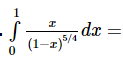Solution:
QUESTION: 5
The equation of the normal to the circle x2 + y2 + 6x + 4y - 3 = 0 at (1, -2) is
Solution:
QUESTION: 6

Two circles x2 + y2 - 2x + 6y + 6 = 0 and x2 + y2 - 5x + 6y + 15 = 0

Solution:
QUESTION: 7

(1 − ω + ω 2 ) (1 − ω 2 + ω 4 ) (1 − ω 4 + ω 8 ) (1 − ω 8 + ω 16)    =

Solution:
QUESTION: 8

If |z₁ + z₂| = |z₁ - z₂|, then the difference of arguments of z₁ and z₂ is

Solution:
QUESTION: 9
The area of the curve |x|+|y|=4 is
Solution:
QUESTION: 10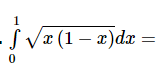Solution:
QUESTION: 11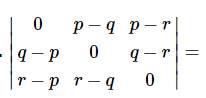Solution:
QUESTION: 12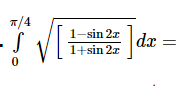Solution:
QUESTION: 13

The singular solution of the differential equation y=px+p3, (p=dy/dx) is :

Solution:
QUESTION: 14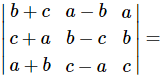Solution:
QUESTION: 15

( d dx )(x2 + cos x)4 =

Solution:

d dx

(x2 + cos x)4
= 4.(x2 + cos x)3 x d dx (x2 + cos x)
= 4(x2 + cos x)3 x (2x - sin x)

QUESTION: 16

The solution of the differential equation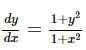Solution: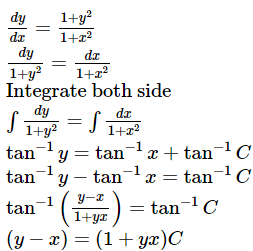QUESTION: 17

The radius of the circle passing through the foci of the ellipse ((x2/16) + (y2/9) = 1), and having its centre (0,3) is

Solution:
QUESTION: 18

(d/dx)[tan⁻1(secx+tanx)]=

Solution:
QUESTION: 19

The tangents to the hyperbola x2 - y2 = 3 are parallel to the st. line 2x + y + 8 = 0 at the following points

Solution:
QUESTION: 20

The equation of the ellipse in the form of ((x2/a2) + (y2/b2) = 1), given the eccentricity to be 2/3 and latus rectum 2/3, is

Solution:
QUESTION: 21

The function f(x) = sin 4x + cos 4x increases if

Solution:

f ′ x > ⇒ 4 sin 3 x . cos x − 4 cos 3 x . sin x > 0

⇒ sin x . cos x sin 2 x − cos 2 x > 0
o r sin 2 x . cos 2 x < 0 o r sin 4 x < 0
∴ π < 4 x < 2 π o r 3 π < 4 x < 4 π
∴ π 4 < x < π 2 o r 3 π 4 < x < π .

QUESTION: 22

The st. line lx + my + n = 0 touches the hyperbola x2/a2- y2/b2 = 1 if

Solution:
QUESTION: 23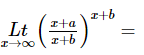Solution:
QUESTION: 24

tan⁻1(x/y) - tan⁻1 (x - y/x + y) is

Solution:
QUESTION: 25

If A and B are two square matrices such that B = -A⁻1 BA, then (A + B)2 =

Solution:
QUESTION: 26

The strength of a beam varies as the product of its breadth b and square of its depth d. A beam cut out of a circular log of radius r would be strong when

Solution: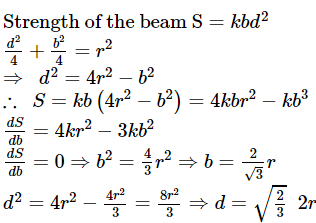QUESTION: 27

|(1)/(2 + i)2 −(1)/(2 − i)2 | =

Solution:
QUESTION: 28

The focus of the parabola x2-8x+2y+7=0 is

Solution:
QUESTION: 29

The axis of the parabola 9y2-16x-12y-57=0 is

Solution:
QUESTION: 30
If nP4:nP3=1:2, then n=
Solution:
QUESTION: 31

Number of divisors of n = 38808 (except 1 and n) is

Solution:

Factorizing the given number, we have
38808 = 23 . 32 . 72 . 11
Therefore the total number of divisors
= (3 + 1) (2 + 1) (1 + 1) - 1 = 71
But this includes the division by the number itself
Hence, the required number of divisors
= 71 - 1 = 70

QUESTION: 32

The number of straight lines that can be formed by joining 20 points of which 4 points are collinear is

Solution:

Number of straight lines = 20C2 - 4C2 + 1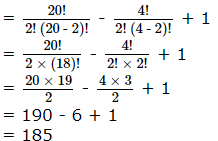QUESTION: 33
In a box, there are 8 red, 7 blue and 6 green balls. One ball is picked up randomly. What is the probability that it is neither red nor green?
Solution:
QUESTION: 34
A bag contains 3 black and 4 white balls. Two balls are drawn one by one at random without replacement the probability that second drawn ball is white, is
Solution:
QUESTION: 35
Three six faced dice are tossed together, then the probability that exactly two of the three numbers are equal, is
Solution:
QUESTION: 36

If b,c and sinB are given such that ∠B is acute and b<c sinB, then

Solution:

When two sides and angle opposite to one of then be given. In this case, the triangle is not always uniquely determined. It is quite possible to have no triangle, one triangle and two triangles with this type of data.
So, it is called an ambiguous case.
Let b, c and B are given parts.
When B is acute and b < c sin B, then there is no triangle
By Cosine Law
b2 = c2 + a2 - 2ac cos B
⇒ a2 - 2ac cos B = b2 - c2
⇒ a2 - 2ac cos B + c2 cos2 B
= b2 - c2 + c2 cos2 B
⇒ (a - c cos B)2 = b2 - c2 (1 - cos2 B)
= b2 - c2 sin2 B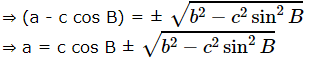This equation helps us to determine a, when b, c and B are being given. We observe that:
When b < c sin B, then a is imaginary and so there is no solution.

QUESTION: 37

If k be the perimeter of the Δ A B C then b cos2 C/ 2 + c cos2 B /2 is equal to

Solution: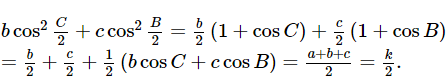QUESTION: 38

If x2+ax+10 = 0 and x2+bx-10=0 have a common rooot,then a2-b2 is equal to

Solution:
QUESTION: 39

The range of values of a so that the equation x3 - 3x + a = 0 has three real and distinct roots is

Solution: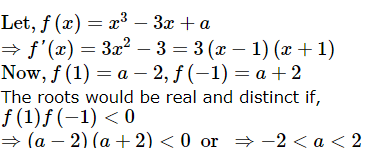⇒ a − 2 a + 2 < 0 or ⇒ − 2 < a < 2
Thus the given equation would have real and distinct roots is a ∈ (-2,2)

QUESTION: 40

If (1 -p) is a root of quadratic equation x2 + px + (1 -p) = 0 then its roots are

Solution:
QUESTION: 41
The number of terms common to two A.P.'s 3, 7, 11, ..., 407 and 2, 9, 16,...., 709 is
Solution:
QUESTION: 42

If a, b, c are in A.P., , mb, c are in G.P.then a, m2b, c are in

Solution:
QUESTION: 43

21/4 41/8 81/16 161/32 ... upto ∞ is equal to

Solution:
QUESTION: 44
The sum of all 2-digit odd numbers is
Solution:
QUESTION: 45
Out of 800 boys in a school, 224 played cricket, 240 played hockey and 336 played basketball. Of the total, 64 played both basketball and hockey; 80 played cricket and basketball and 40 played cricket and hockey; 24 played all the three games. The number of boys who did not play any game is
Solution:
QUESTION: 46
In a city 20 percent of the population travels by car, 50 percent travels by bus and 10 percent travels by both car and bus. Then persons travelling by car or bus is
Solution:
QUESTION: 47
The equation of a line passing through (1,2) and perpendicular to 3x+4y+5=0 is
Solution:
QUESTION: 48
A line L passes through points (1,1) and (2,0) and Line L' passes through [(1/2),0] and perpendicular to L, then the area triangle formed by L, L' and y-axis is
Solution:
QUESTION: 49

If α,β are the roots of x2+ax+b=0, α33=

Solution:
QUESTION: 50

If 2tan2θ=sec2θ, the general value of θ is

Solution:
QUESTION: 51

Consider the equation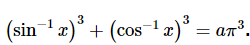3 . The parameter 'a' so that the given equation has a solution which satisfies

Solution:
QUESTION: 52

The sum to infinity of the series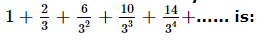Solution:
QUESTION: 53

Let f x be an odd function in the interval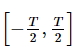with a period T, then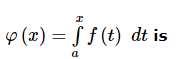Solution:
QUESTION: 54

Let α , β be any two positive values of x for which 2 cos x , | cos x | and 1 − 3 cos 2 x are in G.P., then the minimum value of | α − β | is

Solution:
QUESTION: 55

If ω is cube root of unity, then the value of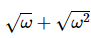is equal to

Solution:
QUESTION: 56

The range of value of β such that (0, β ) lie on or inside the triangle formed by the lines y + 3x + 2 = 0, 3y − 2x − 5 = 0, 4x + x − 14 = 0 is:

Solution:
QUESTION: 57

If the line x cos θ + y sin θ = 2 is the equation of a transverse common tangent to the circles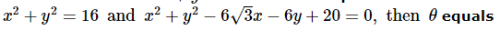Solution:
QUESTION: 58

If A, B and C are three sets such that A ∩ B = A ∩ C and A ∪ B = A ∪ C , then :

Solution:
QUESTION: 59

The solution of the differential equation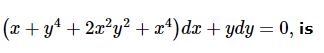Solution:
QUESTION: 60

Let P(3, 2, 6) be a point in space and Q be a point on the line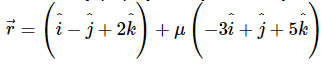Then the value of μ for which the vector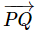is parallel to the plane x - 4y+3z = 1 is

Solution:
QUESTION: 61

If a ‸ , b ‸ and c ‸ are three unit vectors, such that a ‸ + b ‸ + c ‸ is also a unit vector and θ 1 , θ 2 and θ 3 are angles between the vectors a ‸ , b ‸ ; b ‸ , c ‸ and c ‸ , a ‸ respectively then among θ 1 , θ 2 and θ 3

Solution:
QUESTION: 62

If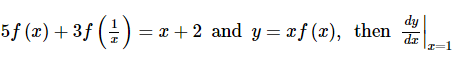is equal to

Solution:
QUESTION: 63

The value of the expression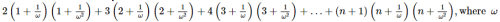, where ω is an imaginary cube roots of unity, is:

Solution:
QUESTION: 64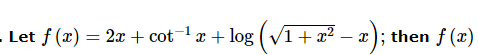Solution:
QUESTION: 65

The set of all value of a ∈ R for which the equation 2x2 − 2(2a+1)x + a(a-1) = 0 has roots α and β satisfying α < a < β is

Solution:
*Multiple options can be correct
QUESTION: 66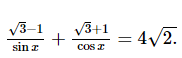About the equation which of the following statements is correct?

Solution:
*Multiple options can be correct
QUESTION: 67

The tangents drawn from (0, 0) to x2 + y2 + 2fy + 2gx + c = 0 are perpendicular if (where c = g2)

Solution:
QUESTION: 68

If P is any point lying on the ellipse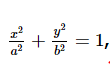, whose foci are S and S'. Let ∠ P S S ′ = α and ∠ P S ′ S = β , then:

Solution:
*Multiple options can be correct
QUESTION: 69

If in a triangle ABC, CD is the angular bisector of the angle ACB then CD is equal to

Solution:
*Multiple options can be correct
QUESTION: 70

If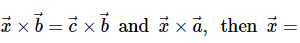Solution:
QUESTION: 71

In a triangle ABC, tan C < 0. Then

Solution:
*Multiple options can be correct
QUESTION: 72

If the equation cx2 + bx - 2a = 0 has no roots and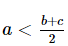then,

Solution:
*Multiple options can be correct
QUESTION: 73

Z 1 , Z 2 , Z 3

correspond to the vertices of an equilateral triangle and | Z 1 − 1 | = | Z 2 − 1 | = | Z 3 − 1 | . Then

Solution:
*Multiple options can be correct
QUESTION: 74

If y = tan x tan 2 x tan 3 x then dy /dx has the value equal to

Solution:
*Multiple options can be correct
QUESTION: 75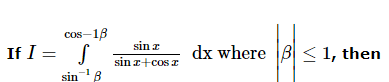Solution:Use Code STAYHOME200 and get INR 200 additional OFF Use Coupon Code